# synthesis

op <- par()
require(zoo)
#>
#> Attaching package: 'zoo'
#> The following objects are masked from 'package:base':
#>
#>     as.Date, as.Date.numeric
library(synthesis)

# 1 Introduction

Synthetic data generation has been widely used not only for its usage in privacy preserving data publishing but also for its capability to support testing of new algorithms or methods. The package of synthesis generates synthetic time series from commonly used statistical models, including linear, nonlinear and chaotic systems. So far, synthesis consists of five groups of statistical models, including linear, nonlinear, dynamical, classification, and state-space systems. The usage of the synthesis package covers the synthetic data generation for variable selection, prediction, and classification and clustering based problems.

# 2 Overview

## 2.1 Linear models

### 2.1.1 Random walk model

The base model of the random walk with drift model (Shumway and Stoffer 2011) is given by, $\begin{equation} \label{eq:2} {{x}_{t}}\text{ =}\delta \text{ +}{{x}_{t-1}}\text{ +}{{w}_{t}} \end{equation}$ where $$t= 1, 2, ..., n$$ and $${{w}_{t}}$$ is Gaussian white noise, $${{w}_{t}}\sim N(0,\sigma_w^{2})$$. The constant $$\delta$$ is known as the drift, and when $$\delta =0$$, it is regarded as a random walk model. The term random walk origins from the fact that when $$\delta =0$$, the value of the time series at time $$t$$ is the value of the series at time $$t-1$$ plus a completely random movement determined by $${{w}_{t}}$$ (Jiang, Sharma, and Johnson 2019). Note that the equation can be formulated as a cumulative sum of white noise variates as follows: $\begin{equation} \label{eq:3} {{x}_{t}}\text{ = }\delta t\text{ + }\sum\limits_{j=1}^{t}{{{w}_{t}}} \end{equation}$ where the drift $$\delta$$ in the model can be seen as the trend of the time series. Therefore, this model is a good proxy for simulating trend, for example, the global temperature data. In the figure below, we show two synthetic time series with and without drift.

### 2.1.2 Autoregressive model

Autoregressive model (AR), as its name suggests, is a regression with lagged values of the variable itself. The general expression of a $$p$$th-order autoregressive process can be written as (Cryer and Chan 2008): $\begin{equation} {{x}_{t}}=c+{{\phi }_{1}}{{x}_{t-1}}+{{\phi }_{2}}{{x}_{t-2}}+\cdots +{{\phi }_{p}}{{x}_{t-p}}+{{\varepsilon }_{t}} \end{equation}$

The following linear AR models are given by Sharma (2000) and included in this package: \begin{align} {{x}_{t}}=0.9{{x}_{t-1}}+0.866{{\varepsilon }_{t}}\\ {{x}_{t}}=0.6{{x}_{t-1}}-0.4{{x}_{t-4}}+{{\varepsilon }_{t}}\\ {{x}_{t}}=0.3{{x}_{t-1}}-0.6{{x}_{t-4}}-0.5{{x}_{t-9}}+{{\varepsilon }_{t}} \end{align}

where $$\epsilon$$ is random Gaussian noise with zero mean and unit standard deviation. For each model, $${{x}_{t}}$$ was arbitrarily initialized and a total number of $$N+500$$ data points were generated. The first 500 points were discarded to reduce any effects from the arbitrary initialization. These AR models are well studied particularly for validating variable selection algorithms (Galelli et al. 2014; Jiang, Sharma, and Johnson 2019, 2020). It should be noted that variants of the random walk and autoregressive models introduced here can also be generated by the arima.sim() from the stats package (R Core Team 2020).

### 2.1.3 Threshold autoregressive model

Here, we show two examples of the two-regime threshold autoregressive (TAR) process(Cryer and Chan 2008) given by Sharma (2000): $\begin{equation} {{x}_{t}}= \begin{cases} -0.9{{x}_{t-3}}+0.1{{\varepsilon }_{t}} & if\text{ }{{x}_{t-3}}\le 0 \\ 0.4{{x}_{t-3}}+0.1{{\varepsilon }_{t}} & if\text{ }{{x}_{t-3}}>0 \end{cases} \end{equation}$

$\begin{equation} {{x}_{t}}= \begin{cases} 0.5{{x}_{t-6}}-0.5{{x}_{t-10}}+0.1{{\varepsilon }_{t}} & if\text{ }{{x}_{t-6}}\le 0 \\ 0.8{{x}_{t-10}}+0.1{{\varepsilon }_{t}} & if\text{ }{{x}_{t-6}}>0 \end{cases} \end{equation}$ where $$\epsilon$$ is random Gaussian noise with zero mean and unit standard deviation. Similarly, for each model, $${{x}_{t}}$$ was arbitrarily initialized and a total number of $$N+500$$ data points were generated. The first 500 points were discarded to reduce any effects from the arbitrary initialization.

set.seed(2021)
sample=500

###Synthetic example - RW model
data.rw <- data.gen.rw(nobs=sample,drift=0.1,sd=1)

plot.ts(data.rw$xd, ylim=c(-35,55), main="Random walk", xlab=NA, ylab=NA, cex.axis=1.5) lines(data.rw$x, col=4); abline(h=0, col=4, lty=2); abline(a=0, b=.1, lty=2)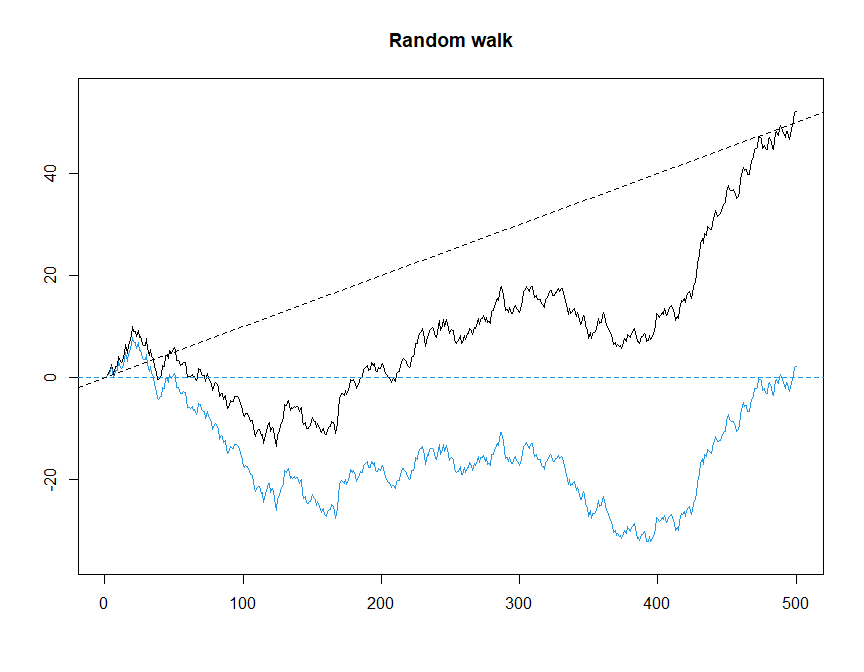Example of Random walk model


###Synthetic example - AR models
ar1 <- data.gen.ar1(nobs=sample)$x ar4 <- data.gen.ar4(nobs=sample)$x
ar9 <- data.gen.ar9(nobs=sample)$x plot.zoo(cbind(ar1,ar4,ar9), col=c("black","red","blue"), ylab=c("AR1","AR4","AR9"), main=NA, xlab=NA, cex.axis=1.5)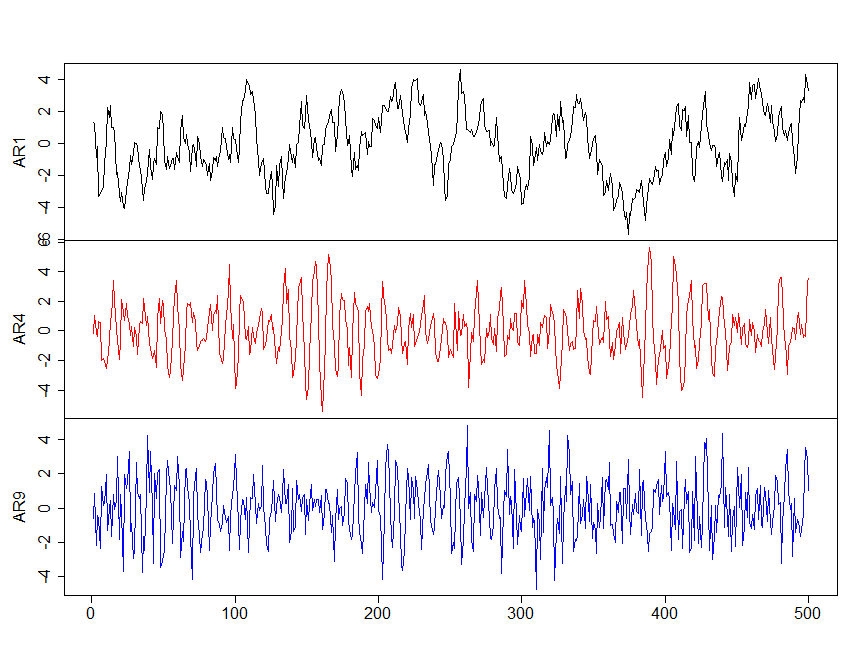Example of Autoregressive models  ###Synthetic example - TAR models # Two TAR models in Sharma (2000) tar1 <- data.gen.tar1(nobs=1000)$x #TAR in Equation (8)
tar2 <- data.gen.tar2(nobs=1000)$x #TAR in Equation (9) # Generalized TAR, an example in Jiang et al. (2020) tar <- data.gen.tar(nobs=1000,ndim=9,phi1=c(0.6,-0.1), phi2=c(-1.1,0),theta=0,d=2,p=2,noise=0.1)$x

plot.zoo(cbind(tar1,tar2,tar), col=c("black","red","blue"), ylab=c("TAR1","TAR2","TAR"),
main=NA, xlab=NA, cex.axis=1.5)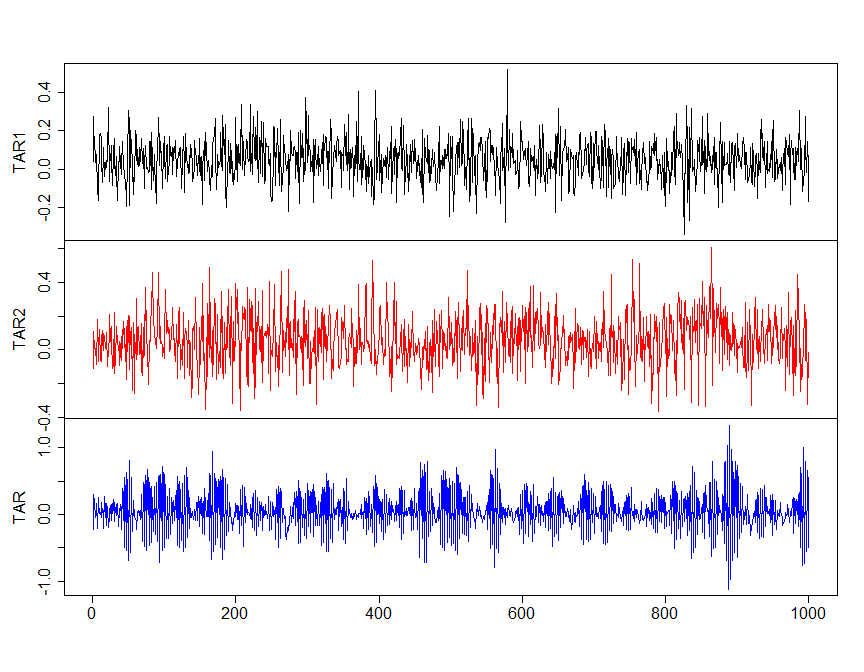Example of Threshold autoregressive models

### 2.1.4 Sinusoidal model

A general form of sinusoidal models can be written as (Shumway and Stoffer 2011):

$\begin{equation} x_t = Acos(2\pi ft + \phi) \end{equation}$ where A is the amplitude, is the phase, and f is the frequency.

set.seed(2021)
sample=500

sw <- data.gen.SW(nobs=sample, freq = 25, A = 2, phi = 0.6*pi, mu=0, sd = 0.1)
plot(sw$t,sw$x, type='o', ylab='Cosines', xlab="t")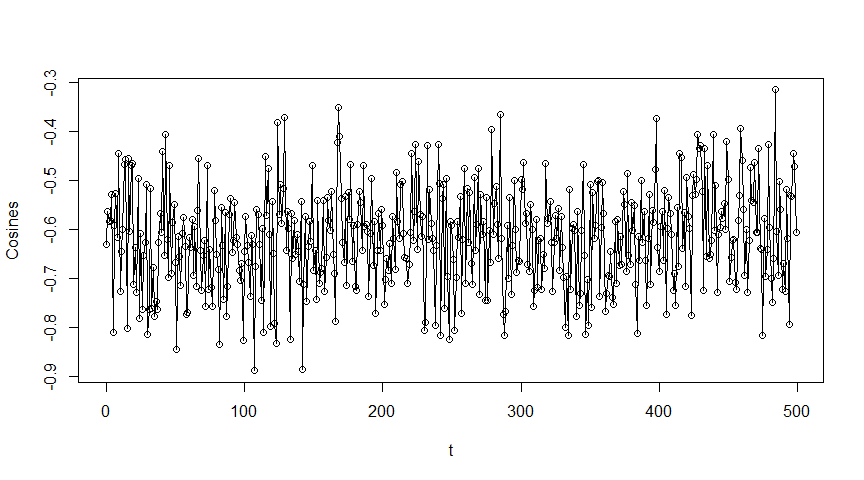Example of Sinusoidal model

## 2.2 Nonlinear systems

### 2.2.1 Hysteresis loop

$\begin{equation} \begin{cases} {{x}_{t}}=a\cos (2\pi ft)+s{{\varepsilon }_{t}} \\ {{y}_{t}}=b\cos {{(2\pi ft)}^{m}}-c\sin {{(2\pi ft)}^{n}}+s{{\varepsilon }_{t}} \end{cases} \end{equation}$ where $$a$$, $$b$$ and $$c$$ are parameters, $$m$$ and $$n$$ are integer numbers, and s is a scaling factor used to alter the levle of noise in the output, which all together specify the classical hysteresis loop (HL). The default HL model datasets was generated from $$y_t$$ with $$f = 25Hz$$, and additional nine candidate predictors were generated with various frequencies. The default values of the system parameters are $$a = 0.8$$, $$b = 0.6$$, and $$c = 0.2$$, which is known to produce a typical form of hysteresis system. As an example, the formulation of the synthetic data from Jiang, Sharma, and Johnson (2020) is shown in the figure below.

### 2.2.2 Friedman

$\begin{equation} y=10\sin (\pi {{x}_{1}}{{x}_{2}})+20\sin {{({{x}_{3}}-0.5)}^{2}}+10{{x}_{4}}+5{{x}_{5}}+s\varepsilon \end{equation}$ where $$s$$ is a scaling factor used to alter the level of noise in the output. Variate, $$x_i$$, is sampled from a uniform distribution, $$x\sim U(0,1)$$, for all $$i = 1, ..., 5$$. In the original formulation, 10-dimension inputs $$x$$ are generated while only first five inputs are relevant with the response. Additionally, datasets can be generated with both zero and various degrees of collinearity. The 10 candidate inputs were generated from correlated uniform variates according to the method by Fackler (1999). In each generated dataset, additional 500 data points were discarded so as to reduce the effect of an arbitrary initialization.

sample=1000
###synthetic example - Hysteresis loop
#Frequency, sampled from a given range
fd <- c(3,5,10,15,25,30,55,70,95)
data.HL <- data.gen.HL(nobs=sample,m=3,n=5,fp=25,fd=fd, sd.x=0, sd.y=0)

plot(data.HL$x,data.HL$dp[,data.HL$true.cpy], xlab="x", ylab = "y", type = "p", cex.axis=1.5,cex.lab=1.5)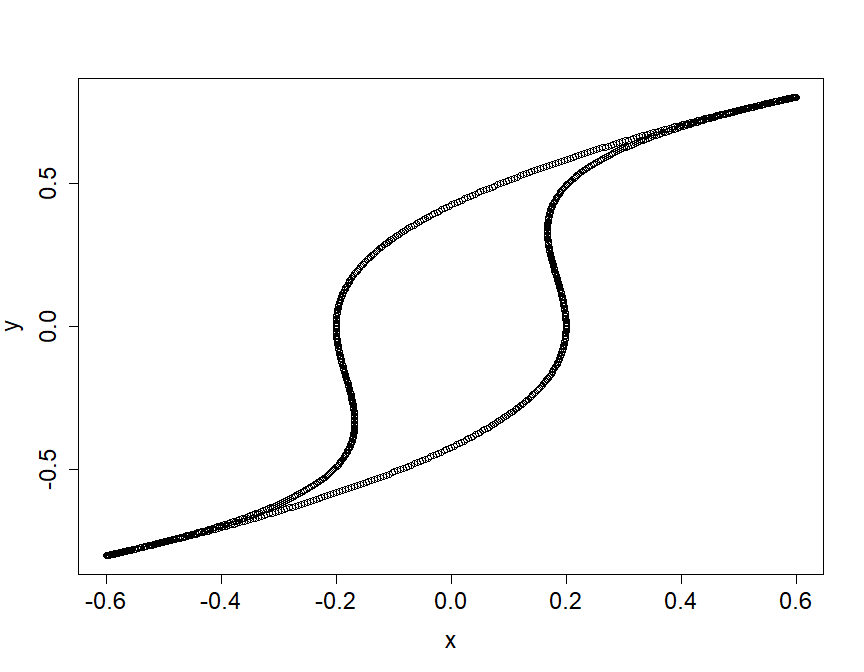Example of Hysteresis loop ###synthetic example - Friedman #Friedman with independent uniform variates data.fm1 <- data.gen.fm1(nobs=sample, ndim = 9, noise = 0) #Friedman with correlated uniform variates data.fm2 <- data.gen.fm2(nobs=sample, ndim = 9, r = 0.6, noise = 0) plot.zoo(cbind(data.fm1$x,data.fm2$x), col=c("red","blue"), main=NA, xlab=NA, ylab=c("Friedman with \n independent uniform variates", "Friedman with \n correlated uniform variates"))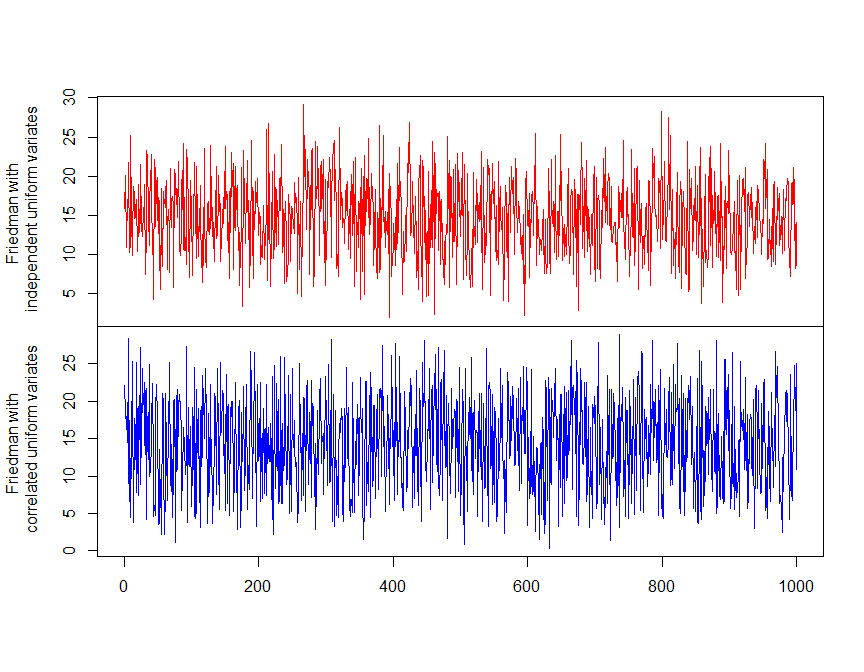Example of Friedman with independent and correlated uniform variates ## 2.3 Dynamic systems ### 2.3.1 Hénon map The Hénon map devised by Hénon (1976) is a two-dimensional map used to illuminate microstructure of strange attractors. $\begin{equation} \begin{cases} {{x}_{n+1}}=1-ax_{n}^{2}+{{\theta }_{n}} \\ {{\theta }_{n+1}}=b{{x}_{n}} \end{cases} \end{equation}$ where $$a$$ and $$b$$ are two parameters. One type of chaotic Hénon maps has values of $$a = 1.4$$ and $$b = 0.3$$. With varying values of $$a$$ and $$b$$, the map may be chaotic, intermittent, or converging to a periodic orbit. The initial condition of this system is randomly generated from a uniform distribution ranging from $$(-0.5, 0.5)$$, and similarly the first 500 points were discarded so as to reduce the effect of an arbitrary initialization. ### 2.3.2 Logistic map The Logistic map can be given as (Strogatz 2000): $\begin{equation} {{x}_{n+1}}=r{{x}_{n}}(1-{{x}_{n}}) \end{equation}$ where $$r$$ represents the growth rate and the value of the control parameter $$r$$ is restricted to the range of $$[0, 4]$$. The initial condition of this system is randomly generated from a uniform distribution ranging from $$(0, 1)$$, and the first 500 points were discarded in order to reduce the effect of an arbitrary initialization. ### 2.3.3 Duffing map A Duffing map, also known as Holmes map, can be expressed as (Dignowity et al. 2013): $\begin{equation} \begin{cases} {{x}_{n+1}}={{\theta }_{n}} \\ {{\theta }_{n+1}}=-\beta {{x}_{n}}+\alpha {{\theta }_{n}}-\theta _{n}^{3} \end{cases} \end{equation}$ ###Synthetic example - Iterated mappings set.seed(2021) par(mfrow=c(1,3), ps=12, cex.lab=1.5, pty="s") sample <- 1000 Henon.map <- data.gen.Henon(nobs = sample, do.plot=TRUE) Logistic.map <- data.gen.Logistic(nobs = sample, do.plot=TRUE) Duffing.map <- data.gen.Duffing(nobs = sample, do.plot=TRUE)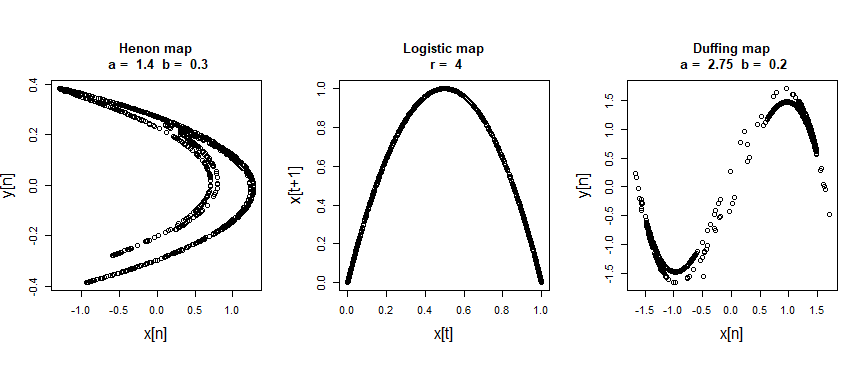Example of Hénon map ### 2.3.4 Rössler system $\begin{equation} \begin{cases} \dot{x}=-y-z, \\ \dot{y}=x+ay, \\ \dot{z}=b+z(x-c). \end{cases} \end{equation}$ The chaotic system depends on three parameters, $$a$$, $$b$$ and $$c$$.The system parameters with values of $$a = 0.2$$, $$b = 0.2$$, and $$c = 5.7$$ can produce a deterministic chaotic time series (Harrington and Van Gorder 2017). In default setting, the time range is fixed from 0 to 50, and a total number of $$N=1000$$ paired observations $$(x_t, y_t, z_t)$$ were generated from an initial condition of $$(-2, -10, 0.2)$$ with no white noise. This generator is also available in the Wavelet System Prediction (WASP) from Jiang et al. (2020). ###Synthetic example - Rossler ts.r <- data.gen.Rossler(a = 0.2, b = 0.2, w = 5.7, start=c(-2, -10, 0.2), time = seq(0, 50, length.out = 5000), s=0) par(mfrow=c(1,2), ps=12, cex.lab=1.5) plot(ts.r$x,ts.r$y, xlab="x",ylab = "y", type = "l") plot(ts.r$x,ts.r$z, xlab="x",ylab = "z", type = "l")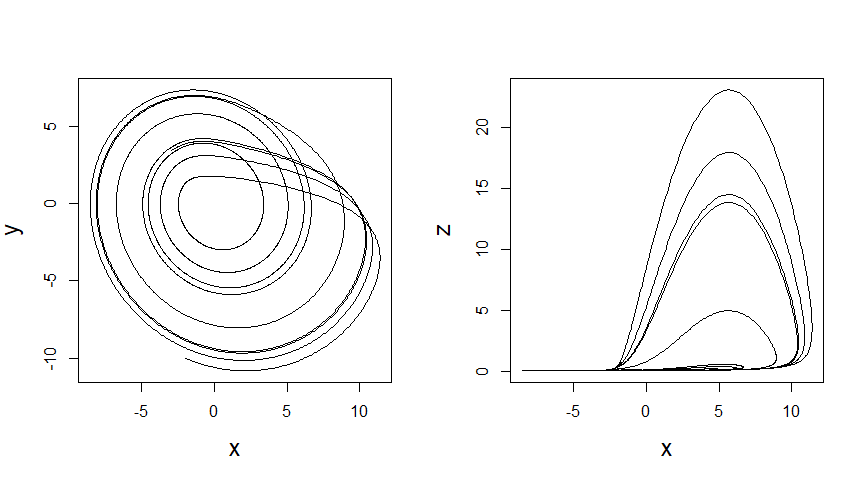Example of Rossler system: Phase portraits in a 2D projection of its state space ### 2.3.5 Lorenz system $\begin{equation} \begin{cases} \dot{x}=\sigma (y-x), \\ \dot{y}=x(\rho -z)-y, \\ \dot{z}=xy-\beta z. \end{cases} \end{equation}$ where $$\sigma$$, $$\rho$$, $$\beta$$>0 are parameters. The default values for the system parameters are $$\sigma=10$$, $$\rho=28$$, $$\beta=8/3$$ and the time range is fixed from 0 to 50, and a total number of $$N=1000$$ paired observations $$(x_t, y_t, z_t)$$ were generated from an initial condition of $$(-13, -14, 47)$$ with no white noise. ###Synthetic example - Lorenz ts.l <- data.gen.Lorenz(sigma = 10, beta = 8/3, rho = 28, start = c(-13, -14, 47), time = seq(0, 50, length.out = 5000), s=0) par(mfrow=c(1,2), ps=12, cex.lab=1.5) plot(ts.l$x,ts.l$y, xlab="x",ylab = "y", type = "l") plot(ts.l$x,ts.l$z, xlab="x",ylab = "z", type = "l")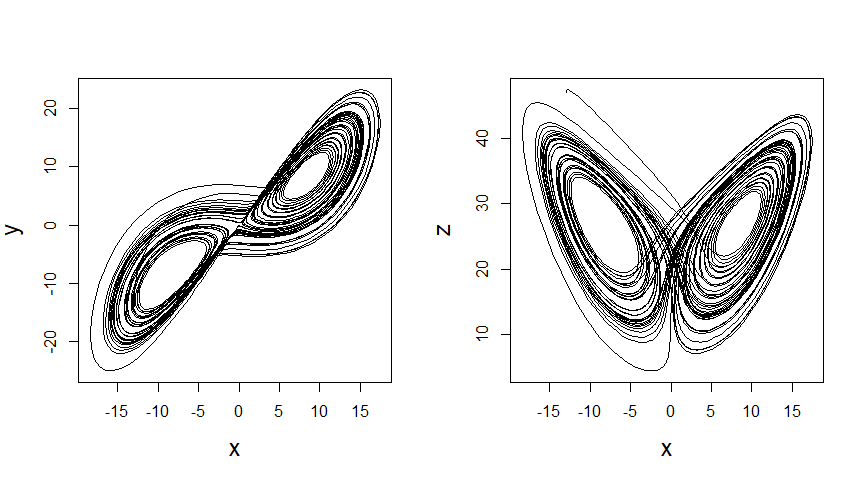Example of Lorenz system: Phase portraits in a 2D projection of its state space ## 2.4 Classification systems ### 2.4.1 Blobs $\begin{equation} p(\mathbf{x}|{{\mu }_{i}},{{\mathbf{\Sigma }}_{i}})=\frac{1}{{{(2\pi )}^{D/2}}|{{\mathbf{\Sigma}}_{i}}{{|}^{1/2}}}\exp \left\{ -\frac{1}{2}{{(\mathbf{x}-{{\mu }_{i}})}^{T}}\;{{\mathbf{\Sigma}}_{i}^{-1}}\;(\mathbf{x}-{{\mu }_{i}}) \right\} \end{equation}$ where $$\mu_i$$ is the mean vector and $$\Sigma_i$$ is covariance matrix. The idea is to place “blobs” of probability mass in the space to cover the data well. ### 2.4.2 Circles $\begin{equation} \begin{matrix} x=a+r\cos t \\ y=b+r\sin t \end{matrix} \end{equation}$ where $$t$$ is a parametric variable in the range of 0 to $$2\pi$$. Gaussian noise can be added to each data point generated by the function of the synthesis package. ### 2.4.3 Spirals $\begin{equation} \begin{matrix} x=r(\varphi )\cos \varphi \\ y=r(\varphi )\sin \varphi \end{matrix} \end{equation}$ where $$r$$ is a monotonic continuous function of angle $$\varphi$$. Examples of datasets generated by these three classification-based functions in the synthesis package are provided below. set.seed(2021) sample=500 par(mfrow=c(1,3), ps=12, cex.lab=1.5, pty="s") Blobs=data.gen.blobs(nobs=sample, features=2, centers=5, sd=1, bbox=c(-10,10), do.plot=TRUE) Circles=data.gen.circles(n = sample, r_vec=c(1,1.5), start=runif(1,-1,1), s=0.1, do.plot=TRUE) Spirals=data.gen.spirals(n = sample, cycles=3, s=0.01, do.plot=TRUE)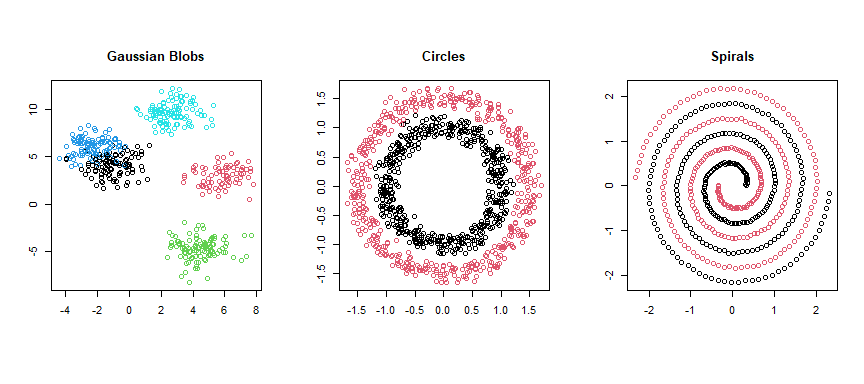Example of Classification system: Blobs, Circles and Spirals ## 2.5 State-space model A state-space model or the dynamic linear model, which was introduced in Kalman (1960) and Kalman and Bucy (1961). The model arose in the space tracking setting, where the state equation defines the motion equations for the position or state of a spacecraft. ### 2.5.1 Linear Gaussian state-space model $\begin{equation} \begin{matrix} x_t = & \phi x_{t-1} + \sigma_v v_t \\ y_t = & x_t + \sigma_e e_t \end{matrix} \end{equation}$ where $$v_t$$ and $$e_t$$ denote independent standard Gaussian random variables, i.e. $$N(0,1)$$. ###Linear Gaussian state-space model data.LGSS <- data.gen.LGSS(theta=c(0.75,1.00,0.10), nobs=500, do.plot = TRUE)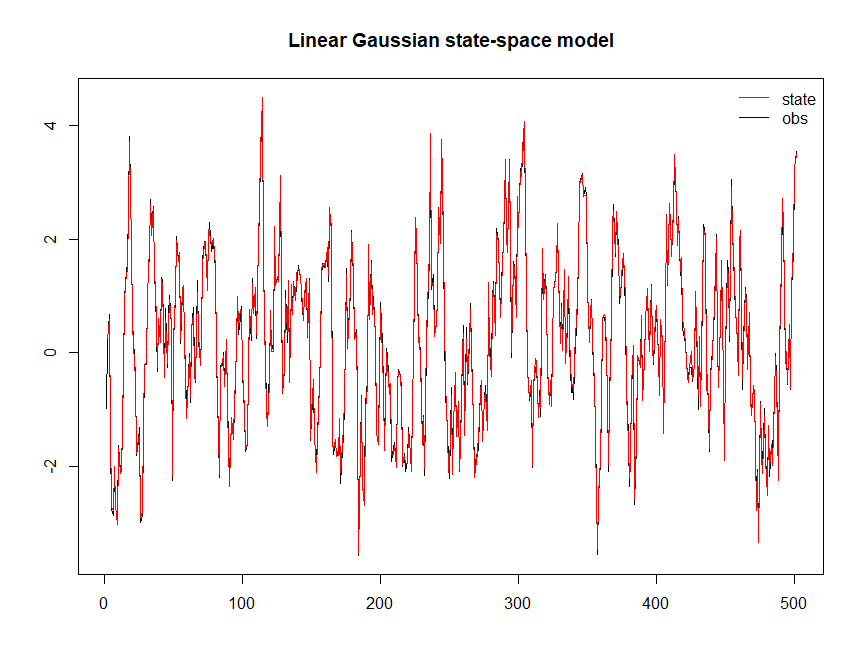Example of linear Gaussian state-space model ## 2.6 Affine error model An affine error model relating measurements to a (geophysical) variable, a standard form used in the triple collocation literature (Zwieback et al. 2012): # Affine error model with 1 true observation and 3 dummy variables data.affine<-data.gen.affine(500) plot.ts(cbind(data.affine$x,data.affine$dp), main="Affine error model")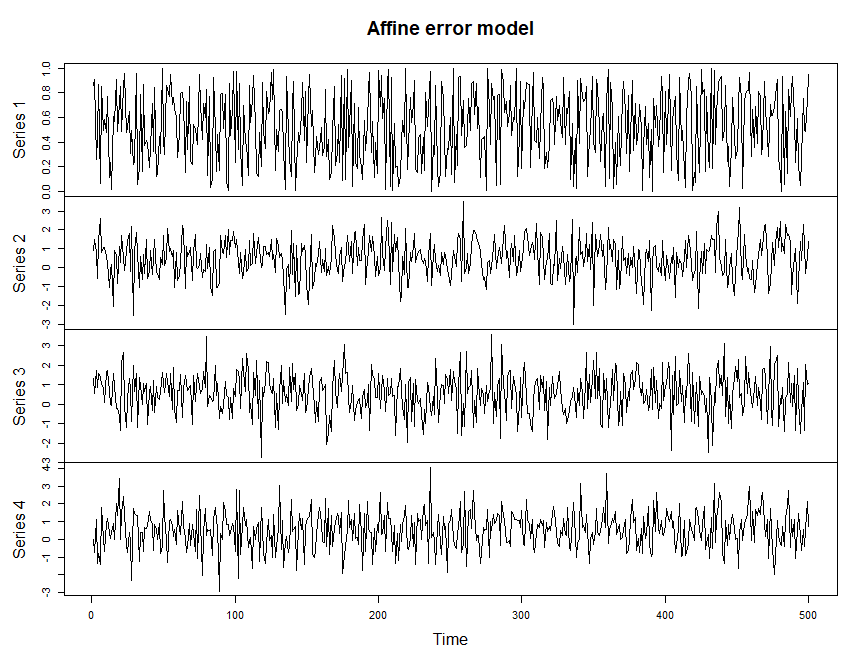Example of the affine error model ## 2.7 Brownian motion models Brownian motion is the random motion of particles suspended in a medium (a liquid or a gas). A geometric Brownian motion (GBM) (also known as exponential Brownian motion) is a continuous-time stochastic process in which the logarithm of the randomly varying quantity follows a Brownian motion (also called a Wiener process) with drift. A fractional Brownian motion (fBm) is a generalization of Brownian motion. Unlike classical Brownian motion, the increments of fBm need not be independent. # Brownian motion models set.seed(100) sample <- 500 par(mfrow=c(1,3), ps=10, cex.lab=1.5, pty="s") data.bm <- data.gen.bm(do.plot = TRUE) data.gbm <- data.gen.gbm(do.plot = TRUE) data.fbm <- data.gen.fbm(do.plot = TRUE)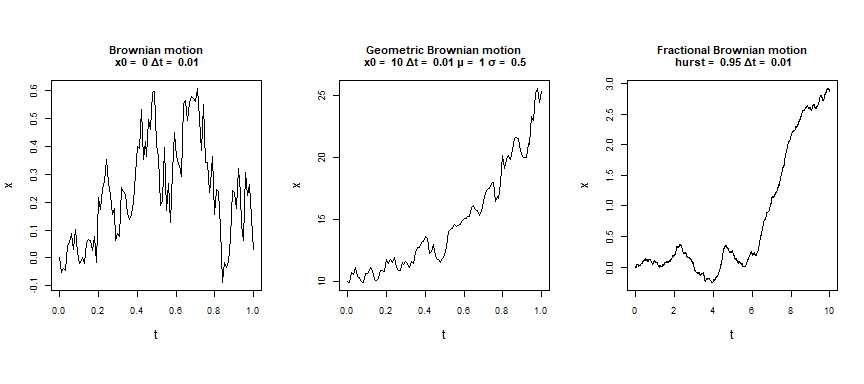Example of Brownian motion models par(op) #> Warning in par(op): graphical parameter "cin" cannot be set #> Warning in par(op): graphical parameter "cra" cannot be set #> Warning in par(op): graphical parameter "csi" cannot be set #> Warning in par(op): graphical parameter "cxy" cannot be set #> Warning in par(op): graphical parameter "din" cannot be set #> Warning in par(op): graphical parameter "page" cannot be set ## 2.8 Build-up and wash-off models In water quality modelling, particulate pollutant buildup and loss (e.g., TSS) from urban and suburban catchments representing a mix of pervious and impervious surfaces has been calculated by using more sophisticated buildup/wash-off (BUWO) models (Wu, Marshall, and Sharma 2019). # Build up and wash off model set.seed(101) sample = 500 #create a gamma shape storm event q<- seq(0,20, length.out=sample) p <- pgamma(q, shape=9, rate =2, lower.tail = T) p <- c(p,p[2:sample]-p[1:(sample-1)]) data.tss<-data.gen.BUWO(sample, k=0.5, a=5, m0=10, q=p) plot.zoo(cbind(p, data.tss$x, data.tss\$y), xlab=NA,
ylab=c("Q","Bulid-up","Wash-off"), main="TSS")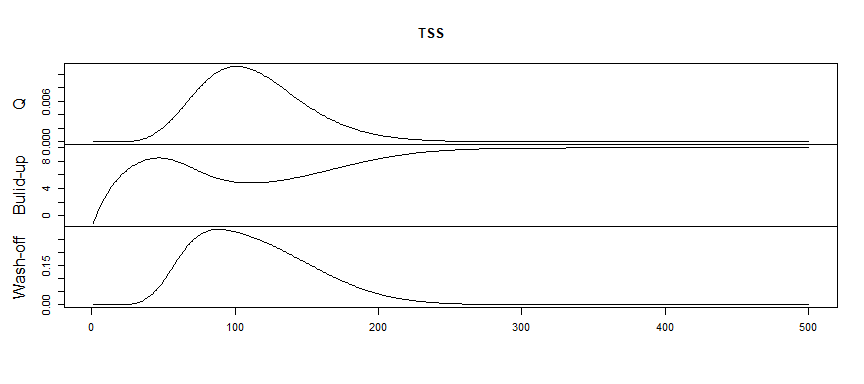Example of build-up and wash-off models

Cryer, Jonathan D., and Kung-Sik Chan. 2008. Time Series Analysis : With Applications in r. Book. New York: Springer New York.
Dignowity, Doreen, Mario Wilson, Piero Rangel-Fonseca, and Vicente Aboites. 2013. “Duffing Spatial Dynamics Induced in a Double Phase-Conjugated Resonator.” Journal Article. Laser Physics 23 (7): 075002.
Fackler, Paul L. 1999. “Generating Correlated Multidimensional Variates.” Journal Article. Department of Agricultural and Resource Economics, North Carolina State University. Http://Www4. Ncsu. Edu/Unity/Users/p/Pfackler/Www/Randcorr. Ps.
Galelli, S., G. B. Humphrey, H. R. Maier, A. Castelletti, G. C. Dandy, and M. S. Gibbs. 2014. “An Evaluation Framework for Input Variable Selection Algorithms for Environmental Data-Driven Models.” Journal Article. Environmental Modelling and Software 62: 33–51. https://doi.org/10.1016/j.envsoft.2014.08.015.
Harrington, Heather A, and Robert A Van Gorder. 2017. “Reduction of Dimension for Nonlinear Dynamical Systems.” Journal Article. Nonlinear Dynamics 88 (1): 715–34.
Hénon, Michel. 1976. “A Two-Dimensional Mapping with a Strange Attractor.” Book Section. In The Theory of Chaotic Attractors, 94–102. New York: Springer.
Jiang, Ze, Md. Mamunur Rashid, Fiona Johnson, and Ashish Sharma. 2020. A wavelet-based tool to modulate variance in predictors: An application to predicting drought anomalies.” Environmental Modelling & Software 135 (October): 104907. https://doi.org/10.1016/j.envsoft.2020.104907.
Jiang, Ze, Ashish Sharma, and Fiona Johnson. 2019. “Assessing the Sensitivity of Hydro-Climatological Change Detection Methods to Model Uncertainty and Bias.” Journal Article. Advances in Water Resources 134: 103430. https://doi.org/https://doi.org/10.1016/j.advwatres.2019.103430.
———. 2020. “Refining Predictor Spectral Representation Using Wavelet Theory for Improved Natural System Modeling.” Journal Article. Water Resources Research 56 (3): e2019WR026962. https://doi.org/https://doi.org/10.1029/2019WR026962.
Kalman, Rudolph E. 1960. “A New Approach to Linear Filtering and Prediction Problems.”
Kalman, Rudolph E., and Richard S. Bucy. 1961. “New Results in Linear Filtering and Prediction Theory.”
R Core Team. 2020. R: A Language and Environment for Statistical Computing. Vienna, Austria: R Foundation for Statistical Computing. https://www.R-project.org/.
Sharma, Ashish. 2000. “Seasonal to Interannual Rainfall Probabilistic Forecasts for Improved Water Supply Management: Part 1 — a Strategy for System Predictor Identification.” Journal Article. Journal of Hydrology 239 (1): 232–39. https://doi.org/https://doi.org/10.1016/S0022-1694(00)00346-2.
Shumway, Robert H, and David S Stoffer. 2011. “Characteristics of Time Series.” Book Section. In Time Series Analysis and Its Applications, 8–14. New York : Springer.
Strogatz, Steven H. 2000. Nonlinear Dynamics and Chaos : With Applications to Physics, Biology, Chemistry, and Engineering. Book. 1st pbk print. Cambridge, MA: Cambridge, MA : Westview Press.
Wu, Xia, Lucy Marshall, and Ashish Sharma. 2019. The influence of data transformations in simulating Total Suspended Solids using Bayesian inference.” Journal Article. Environmental Modelling & Software 121: 104493. https://doi.org/https://doi.org/10.1016/j.envsoft.2019.104493.
Zwieback, S., K. Scipal, W. Dorigo, and W. Wagner. 2012. Structural and statistical properties of the collocation technique for error characterization.” Nonlinear Processes in Geophysics 19 (1): 69–80. https://doi.org/10.5194/npg-19-69-2012.